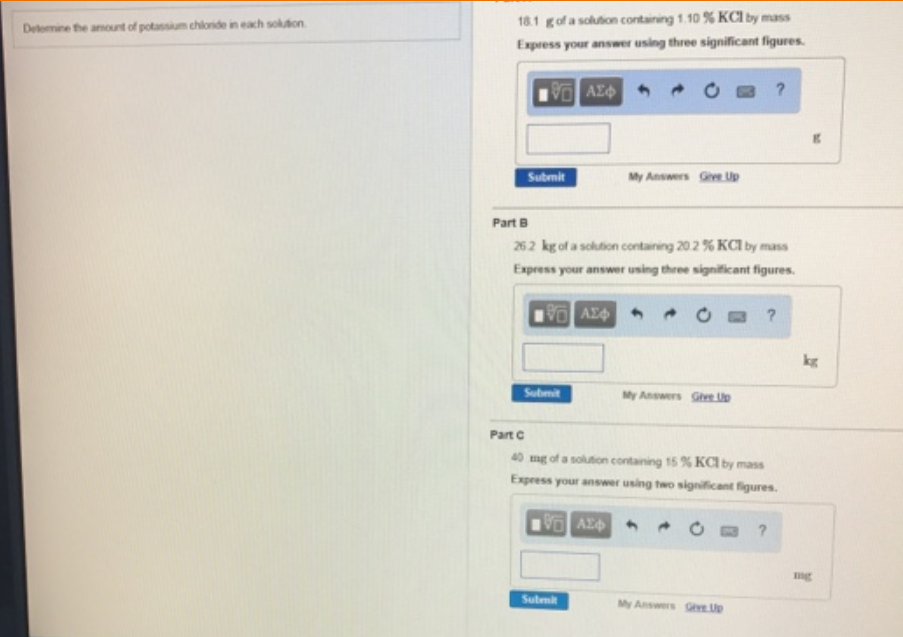# Problem: Determine amount of potassium chloride in each solution.A. 18.1 g of a solution containing 1.10% KCl by mass. Express your answer using three significant figures. B. 26.2 kg of a solution containing 20.2% KCl by mass. Express your answer using three significant figures.C. 40 mg solution containing 15% KCI by mass.  Express your answer using two significant figures.

🤓 Based on our data, we think this question is relevant for Professor Person's class at CSU.

###### FREE Expert Solution###### Problem Details

Determine amount of potassium chloride in each solution.

A. 18.1 g of a solution containing 1.10% KCl by mass. Express your answer using three significant figures.

B. 26.2 kg of a solution containing 20.2% KCl by mass. Express your answer using three significant figures.

C. 40 mg solution containing 15% KCI by mass.  Express your answer using two significant figures.